### Where and How to Write Formulas

ChaosPro has an IDE (Integrated Development Environment) in which you can see all formulas, all formula types and write your own formulas.

Formulas of the same type are grouped in files having specific extensions. Normally different formula authors use different file names: For example you can find several iteration formulas in a file called “ChaosPro.cfm”.
Basically you could write formulas using your favorite editor. But then of course you do not only want to write them, you may wish to compile the formula to see whether there are some errors, and then you may wish to actually use the formula. This cannot be done from outside ChaosPro, so using an external editor may be useful only for very special purposes (formula reorganization, enhanced search-and-replace operations, etc.). Ok, all I wanted to say is that the formula files are ASCII files which can be modified using a standard editor.

Warning: Never try to access the same formula from ChaosPro and from an external program! You never know when ChaosPro will overwrite the formula file using its old formula version. In other words: If you expand a formula file in ChaosPro’s IDE in order to see the formulas in it, then ChaosPro will read the formula file, parse it and display all formulas. Now the contents of the complete file is in ChaosPro’s very own memory. If you now modify the formula file from outside, ChaosPro won’t care about it: It has the formulas in memory. Even worse, as soon as you collapse the formulas in the IDE ChaosPro will take its own (perhaps old) formula versions, create the formula file from scratch by simply appending the different formulas and write the file to hard disk. It will overwrite the complete file. ChaosPro won’t notice any changes made to the file from any external program!

If you start ChaosPro, most probably the IDE (compiler window) will be closed. Please open it by selecting “Menu/ Windows/ Compiler”. You will see a window like that: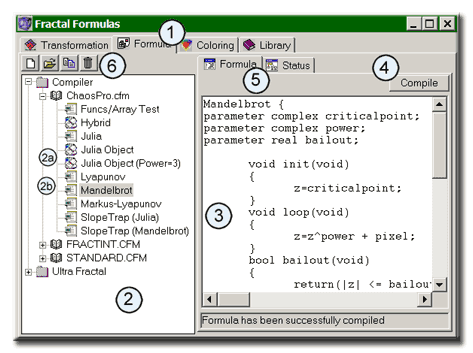The formula compiler in ChaosPro supports four “types” of formulas: Transformations, formulas, colorings and libraries. You can choose the formula type by using the tab (1) at the top of the window.

The left side (2) displays the complete directory structure starting at special directories which you can specify (Compiler search path). ChaosPro will scan the directories for files of the corresponding (formula) type, i.e. for files having the correct extension.
You then can expand a formula file to see the formulas in it.
The icon to the left of each entry has a special meaning:

•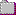– This icon represents a directory on your computer. ChaosPro recursively scans the directory tree below the compiler path and displays the tree.
•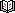– This icon represents a formula file on your computer. A formula file contains a collection of formulas of the same type. ChaosPro recognizes the formula type contained in a file by looking at the file extension.
•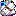(see 2a) – This icon represents a formula inside a formula file written for fractal type “Quaternion”. There is a slight difference in formulas for complex and formulas for quaternion numbers.
•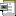(see 2b) – This icon represents a formula inside a formula file written for fractal type “Escapetime”. By looking at the icon you can determine whether you can assign that formula to your fractal.

If you open a formula file, you will see all formulas contained in that specific file.

As soon as you select a formula, the right side of the window (3) will display the formula itself, ready to be modified.

After you are finished modifying the formula, you must press the “Compile”-Button (4). The formula will be compiled and all calculated fractals using that formula will be notified about that: They completely restart fractal calculation based on the new formula.

If you want to assign a formula to a fractal (Escapetime or Quaternion) simply double click onto the formula name in (2) and the currently active fractal gets this formula assigned.

Perhaps you are interested in some statistics of the formula? The “Status”-tab (5) will display all variables and parameters, it will show you how often each variable is referenced (i.e. read), and how often a value gets written to. Using that information you can easily detect unused variables. On the other hand, if you are not interested in such statistical information, simply ignore that page. You cannot adjust anything, its sole purpose is informational.

The toolbar buttons (6) offer some commands: New, Open, Save formula, Save file (and all formulas), Duplicate and Delete.

You can assign comments to each formula file (note: formula file, not formulas!) by selecting the formula file itself (for example “standard.cfm”). The right side then will display the comment for this file. This way you can write some additional comments (for example copyright, how the formulas should be used, some general hints, or whatever).

Please note that there is a context menu: Simply press the right mouse button to access it.

Ok, now lets add a new formula:

Select a formula file, then press the right mouse button over area (2) and choose “New”. A formula called “New_Formula” gets created. It contains the following text:

`  New_Formula  {  parameter complex perturb;    	void init(void)  	{  		z=perturb;  	}  	void loop(void)  	{  		z=z*z+pixel;  	}  	bool bailout(void)  	{  		return(|z|<16);  	}  	void description(void)  	{  		this.title="New_Formula";  		this.center=(1,3);  		this.angle=0;  		this.maxiter=250;  		this.periodicity=0;  		this.helpfile="http://www.chaospro.de/";  		perturb.caption="Perturberation";  		perturb.default=(0,0);  		perturb.hint="Start value for iteration, also called perturberation";  	}  }  `

The syntax of each formula is similar to C/C++ or Java. In C++/Java-syntax I would say, that each formula simply is a class file having a specific set of methods (the methods depend on the formula type). Comments start either with “//” and last upto the end of the line, or they start with a “/*” and end with a “*/”. All text contained between these characters is considered to be comment (i.e. just the same as in C++ or Java).

The above formula consists of four methods (or functions or member functions, just as you like):

• void init(void) : This method gets called for each pixel at the start of the iteration process
• void loop(void) : This method defines the iteration formula
• bool bailout(void) : The method “loop” gets called as long as “bailout” returns true
• void description(void): This member function lets you specify names and default values for the formula and the parameters.

This default formula has one parameter called “perturb”. The type of this parameter is “complex”. Unlike normal programming languages the variable type “complex” is a native data type. The keyword “parameter” is specific to ChaosPro: The compiler lets you specify two types of variables:

• Variables, which you simply use inside the formula: You initialize them, you read from them, you assign values to them.
• Variables, whose initial values should be adjustable to the end user, i.e. parameters. Parameters get defined by the keyw
ord “parameter”. All those parameters can be changed by the user in the parameter window of the fractal (Escapetime or Quaternion). You can write to parameters, although it is not recommended: If you do not write to a parameter, then ChaosPro can assume it to be constant and thus can perform optimizations. If you write to a parameter, then ChaosPro won’t perform optimizations on it.

The whole formula thus reads as follows (it calculates the standard Mandelbrot set):

For each pixel in the fractal window:

Initialize z with the parameter “perturb” which the user has specified

Iterate:

Calculate z*z+pixel

Check whether |z|<16, if not, then stop

Check whether the maximum number of iterations has been reached

Continue with next iteration

So one member function is left: description

Imagine the user in front of the parameter window. The user is prompted to change the parameter “perturb” of formula “New_Formula” to some value. Nice. Indeed. The user knows nothing about the formula, nothing about the meaning of “perturb”, most probably the user does not even care about the definition of the “formula”: He simply wants to calculate a fractal. So there must be some way to specify meaningful labels for the end user, some hints and default values. And that’s the purpose of the member function “description”: “perturb.caption” specifies the label of the complex parameter, and the user only sees that label and not “perturb”. Moreover, “perturb.hint” specifies a hint which will appear if the user moves the mouse over the parameter.

Ok, that’s all for now. The next chapters will describe in more detail the different sections and features of the compiler.